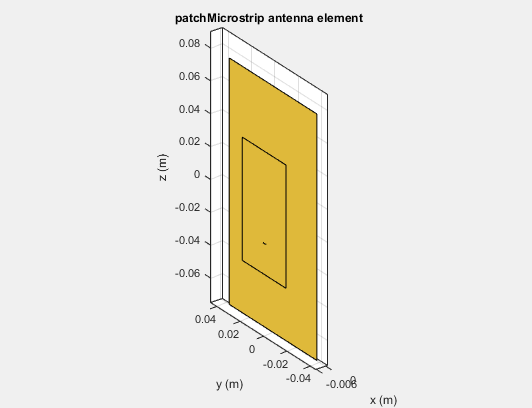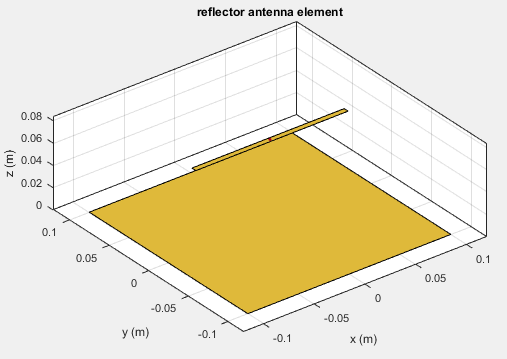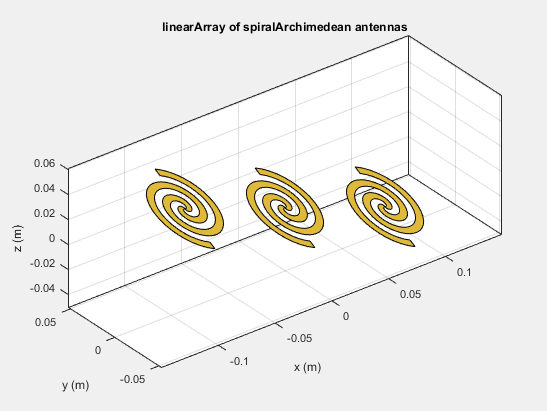## Rotate Antennas and Arrays

To rotate antenna elements in Antenna Toolbox™, use the `Tilt` and `TiltAxis` properties. The `Tilt` property specifies the angles of rotation of the antenna in degrees. The `TiltAxis` property specifies the one or more axes of rotation (X, Y, Z) of the antenna.

### Rotate Single Antenna Element

Create a patch antenna. By default, this antenna is on the X-Y plane.

```patch = patchMicrostrip; show(patch)```To make the antenna lie on the Y-Z plane, rotate it by 90 degrees about the Y-axis. The rotation follows the standard right-hand rule.

```patch.TiltAxis ="Y"; patch.Tilt = 90; show(patch)```The patch lies behind the ground plane and is not visible. To make the patch visible change the `TiltAxis` property to ```[0 -1 0]```. The `Tilt` property is still `90`, but the axis of rotation is now the Y-axis. The negative number in the `TiltAxis` vector determines the direction of rotation about the Y-axis as per the right-hand rule.

```patch.TiltAxis = [0 -1 0]; show(patch) ```### Rotate Single Antenna Element About Multiple Axes

Create a dipole antenna. Change the direction of rotation of the antenna with two rotations simultaneously. Rotate the antenna by `90` degrees about the axis specified by `[0 1 0]` and rotate it by `90` degree about the axis specified by ```[0 1 1]```.

`d = dipole(Tilt=[90 90], TiltAxis=[0 1 0; 0 1 1])`
```dipole with properties: Length: 2 Width: 0.1000 FeedOffset: 0 Tilt: [90 90] TiltAxis: [2x3 double] ```
`show(d)`### Rotate Dipole-Backed Reflector

The `Tilt` and `TiltAxis` properties are available for dipole and reflector elements. Use these properties if you want to model an antenna parallel or perpendicular to the ground plane.

Create a reflector element. By default, the dipole is parallel to the reflector element.

```r = reflector; show(r)```The dipole is the exciter element of the reflector. View its properties.

```r.Exciter; ```
``` dipole with properties: Length: 0.1500 Width: 0.0050 FeedOffset: 0 Tilt: 90 TiltAxis: [0 1 0]```

Based on the `Tilt` and `TiltAxis` properties the dipole element is rotated by 90 degrees about the y-axis and is parallel to the X-Y plane. To make the dipole perpendicular to the X-Y plane, change the `Tilt` property of the dipole to `0` degrees.

```r.Exciter.Tilt = 0; show(r)```Rotating the reflector element rotates the entire structure by the specified angle. To rotate the complete antenna, use the `Tilt` property of the reflector object. For example, rotate the reflector by 90 degrees about the X-axis. According to the right-hand rule, the reflector now lies in the X-Z plane with the dipole.

```r = reflector; r.Tilt = 90; show(r)```### Rotate Antenna Array

Create an array of Archimedean spirals with the whole array rotated at 30 degrees about the X-axis and Y-axis. First create one spiral antenna.

```s = spiralArchimedean; l = linearArray(Element=s, ElementSpacing=0.1,... NumElements=3, Tilt=30, TiltAxis=[1 1 0]); show(l)```